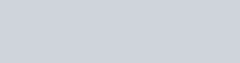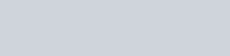Solve the following system of equations graphically.
Question:

Solve the following system of equations graphically.

$2 x-3 y+6=0$

$2 x+3 y-18=0$

Also, find the area of the region bounded by these two lines and y-axis.

Solution:

The given equations are:

$2 x-3 y+6=0$$\ldots \ldots . .(i) 2 x+3 y-18=0$$\ldots \ldots . .(i i)$

Putting $x=0$ in equation (i) we get:

$\Rightarrow 2 \times 0-3 y=-6$

$\Rightarrow y=2$

$x=0, y=2$

Putting $y=0$ in equation (i) we get:

$\Rightarrow 2 x-3 \times 0=-6$

$\Rightarrow x=-3$

$x=-3, \quad y=0$

Use the following table to draw the graphDraw the graph by plotting the two points $A(0,2), B(-3,0)$ from table.$2 x+3 y-18=0$.(ii)

Putting $x=0$ in equation $(i i)$ we get:

$\Rightarrow 2 \times 0+3 y=18$

$\Rightarrow y=6$

$x=0, \quad y=6$

Putting $y=0$ in equation $(i i)$, we get:

$\Rightarrow 2 x+3 \times 0=18$

$\Rightarrow x=9$

$x=9, \quad y=0$

Use the following table to draw the graph.Draw the graph by plotting the two points $C(0,6), D(9,0)$ from table.

The two lines intersect at $P(3,4)$.

Hence $x=3, y=4$ is the solution of the given equations.

The area enclosed by the lines represented by the given equations and the $y$-axis

Now,

$\Rightarrow$ Required area $=$ Area of PCA

$\Rightarrow$ Required area $=1 / 2($ base $\times$ height $)$

$\Rightarrow$ Required area $=1 / 2(C A \times P M)$

$\Rightarrow$ Required area $=1 / 2(4 \times 3)$ sq.units

Hence the required area is $6 \mathrm{sq}$. units